# please answer 5 and 6 5.) (8 pts.) Sketch the solid R in 3D-Space whose volume...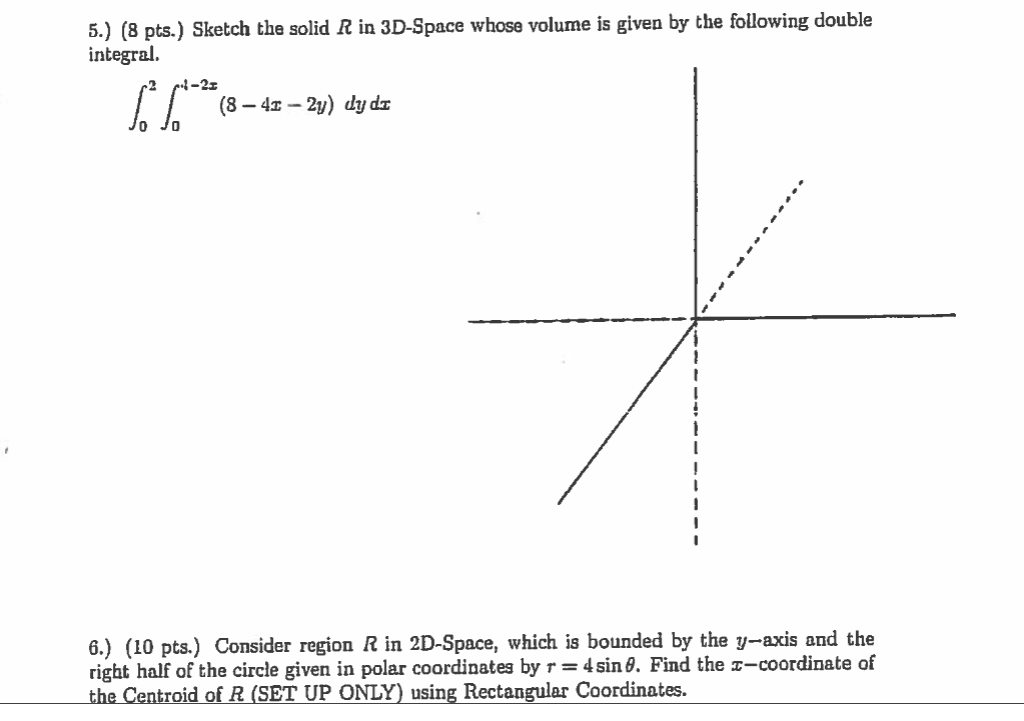5.) (8 pts.) Sketch the solid R in 3D-Space whose volume is given by the following double integral. (8 - 41 -2y) dy dz Jo Jo 6.) (10 pts.) Consider region R in 2D-Space, which is bounded by the y-axis and the right half of the circle given in polar coordinates by s = 4 sin 8. Find the I-coordinate of the Centroid of R (SET UP ONLY) using Rectangular Coordinates.

AS PER CHEGG POLICY, ONLY FIRST QUESTION IS TO BE ANSWERED. I HAVE SOLVED BOTH, BUT FULL EXPLANATION ARE NOT PROVIDED FOR SECOND. PLEASE DON'T MIND!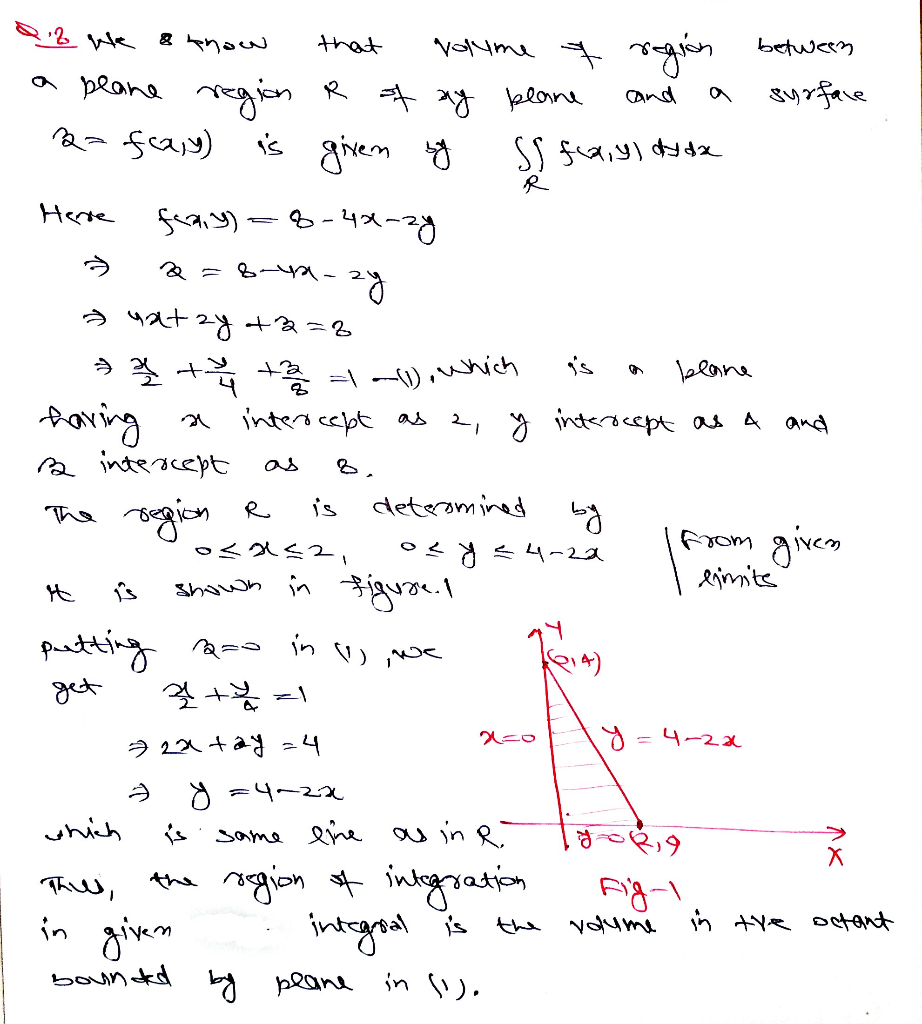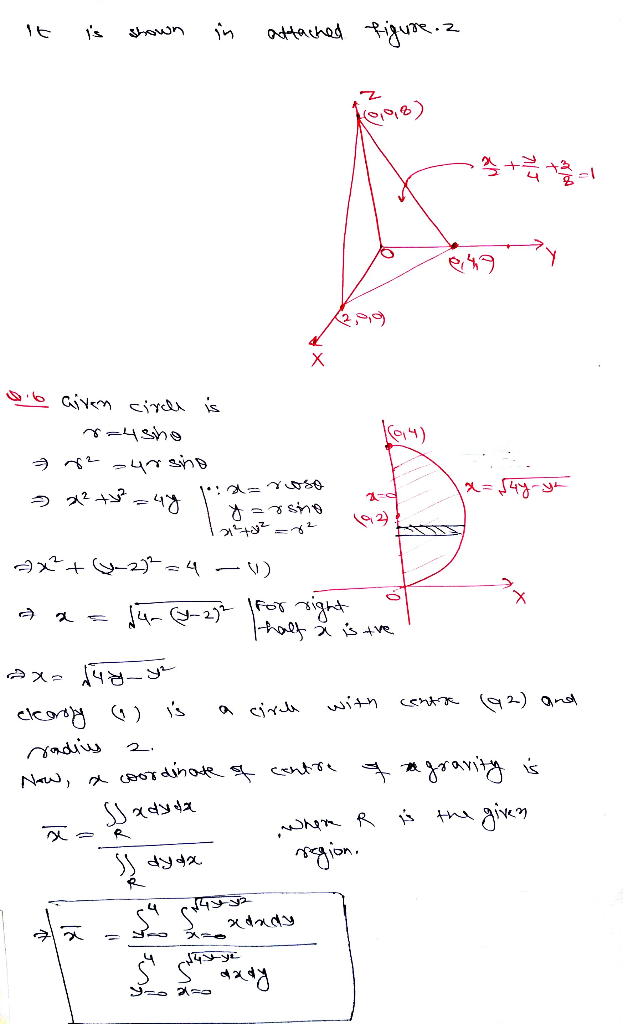#### Earn Coin

Coins can be redeemed for fabulous gifts.

Similar Homework Help Questions
• ### please show complete work 25) Use a triple integral in the coordinate system of your choice to find the volume of the s...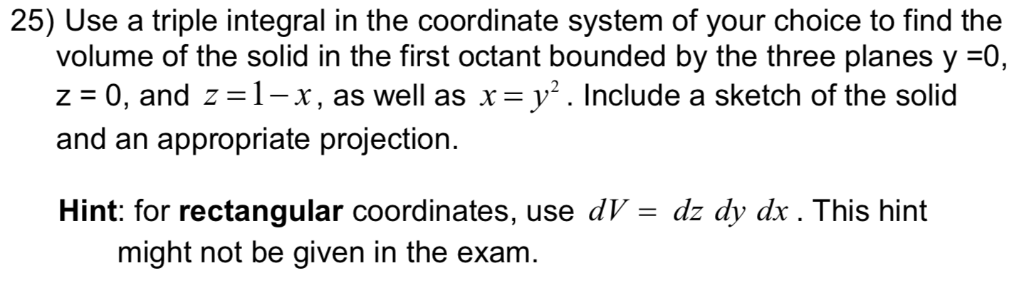please show complete work 25) Use a triple integral in the coordinate system of your choice to find the volume of the solid in the first octant bounded by the three planes y =0 z 0, and z 1-x x y2. Include a sketch of the solid as well as appropriate projection and an Hint: for rectangular coordinates, use dV might not be given in the exam dz dy dx. This hint 25) Use a triple integral in the coordinate...

• ### 6. (4 pts) Consider the double integral∫R(x2+y)dA=∫10∫y−y(x2+y)dxdy+∫√21∫√2−y2−√2−y2(x2+y)dxdy.(a) Sketch the region of integration R in Figure 3.(b)...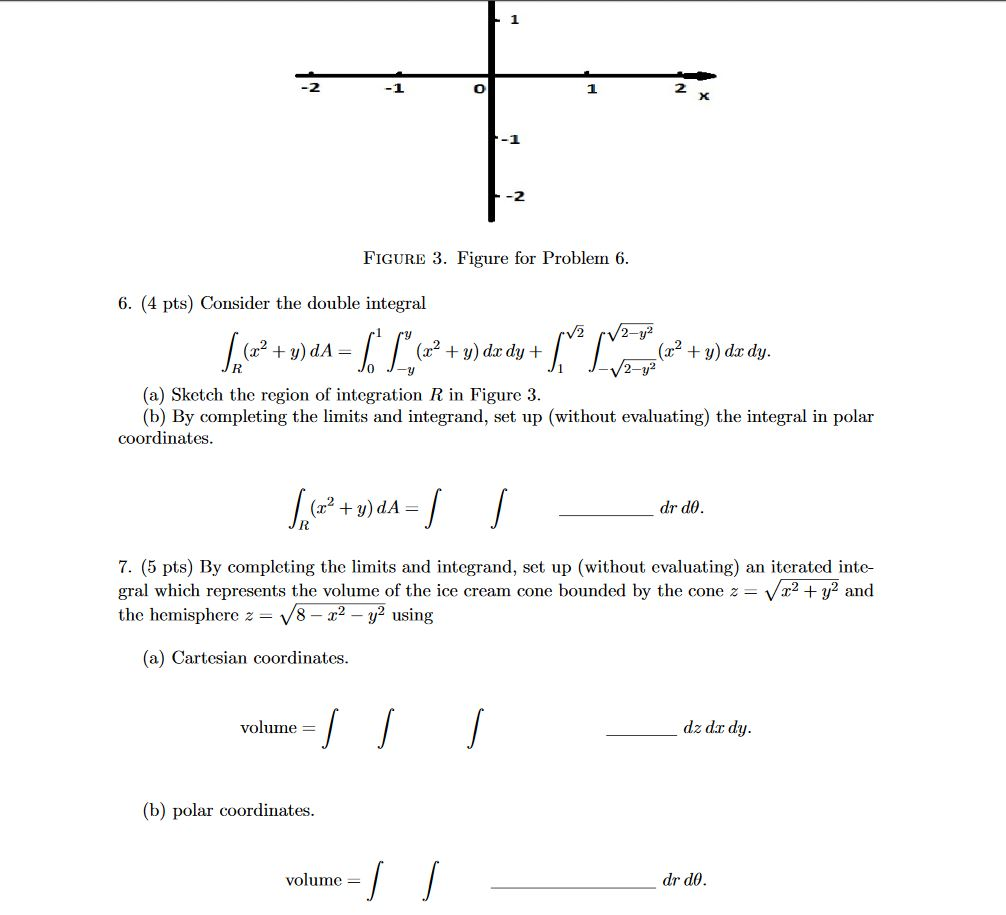6. (4 pts) Consider the double integral∫R(x2+y)dA=∫10∫y−y(x2+y)dxdy+∫√21∫√2−y2−√2−y2(x2+y)dxdy.(a) Sketch the region of integration R in Figure 3.(b) By completing the limits and integrand, set up (without evaluating) the integral in polar coordinates.∫R(x2+y)dA=∫∫drdθ.7. (5 pts) By completing the limits and integrand, set up (without evaluating) an iterated inte-gral which represents the volume of the ice cream cone bounded by the cone z=√x2+y2andthe hemisphere z=√8−x2−y2using(a) Cartesian coordinates.volume =∫∫∫dz dxdy.(b) polar coordinates.volume =∫∫drdθ. -1 -2 FIGURE 3. Figure for Problem 6. 6. (4 pts)...

• ### 6. (12pts) Consider the solid that is above the xy-plane, bounded above by =/4-x-y and below by +y a. Sketch the so...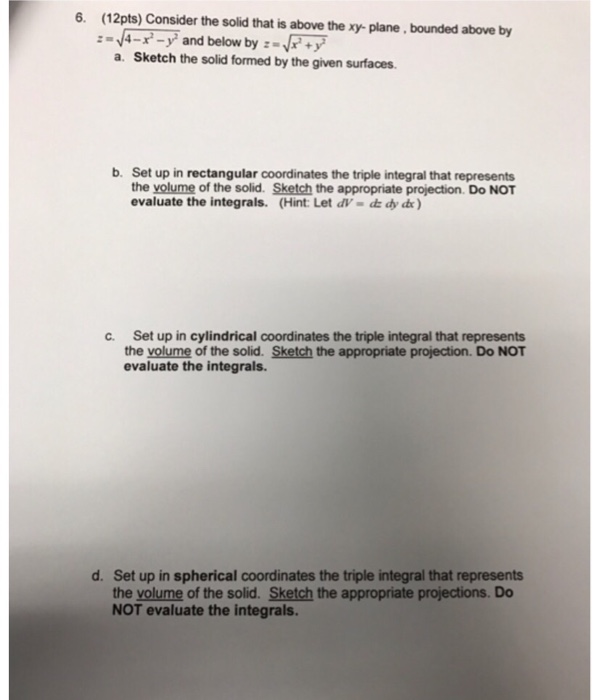6. (12pts) Consider the solid that is above the xy-plane, bounded above by =/4-x-y and below by +y a. Sketch the solid formed by the given surfaces b. Set up in rectangular coordinates the triple integral that represents the yolume of the solid. Sketch the appropriate projection. Do NOT evaluate the integrals. (Hint: Let dV- d dy de) c. Set up in cylindrical coordinates the triple integral that represents the volume of the solid. Sketch the appropriate projection. Do NOT...

• ### 4,5 Sketch the solid whose volume is given by the iterated integral. 2-22 4.  5...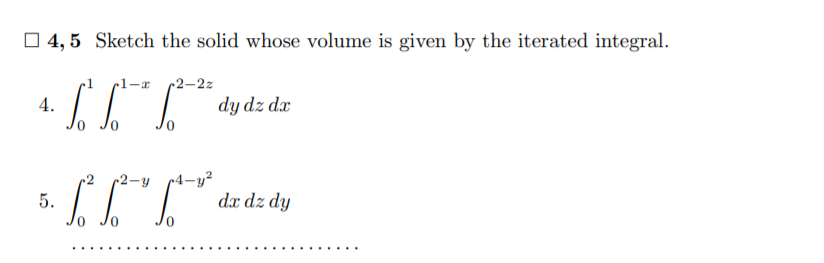4,5 Sketch the solid whose volume is given by the iterated integral. 2-22 4.  5 dydz de c2-y 5. (IT dx dz dy

• ### 6. (4 pts) Consider the double integral∫R(x2+y)dA=∫10∫y−y(x2+y)dxdy+∫√21∫√2−y2−√2−y2(x2+y)dxdy.(a) Sketch the region of integrationRin Figure 3.(b) By completing...6. (4 pts) Consider the double integral∫R(x2+y)dA=∫10∫y−y(x2+y)dxdy+∫√21∫√2−y2−√2−y2(x2+y)dxdy.(a) Sketch the region of integrationRin Figure 3.(b) By completing the limits and integrand, set up (without evaluating) the integral in polar coordinates. -1 -2 FIGURE 3. Figure for Problem 6. 6. (4 pts) Consider the double integral V2 /2-y² + = (x2 + y) dx dy + + y) do dy. 2-y2 (a) Sketch the region of integration R in Figure 3. (b) By completing the limits and integrand, set up (without evaluating)...

• ### Sketch the solid whose volume is given by the iterated integral. 6*6*15 (5 - x -...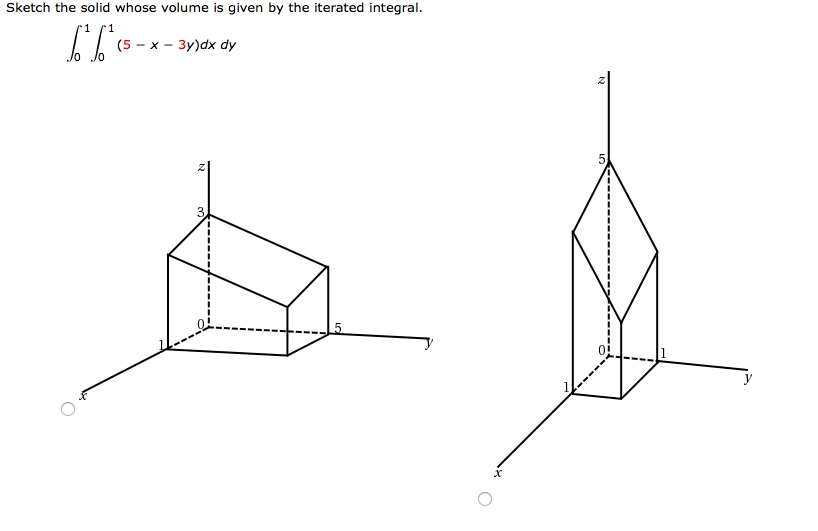Sketch the solid whose volume is given by the iterated integral. 6*6*15 (5 - x - 3y)dx dy 2 z 5 2 z WebAssign Plot

• ### please show all work in clean and legible handwriting with all labels and steps that is properly explained for PROBLEMS #1, 2, 3, AND 4. Any incorrect answers and not solving all 4 problems...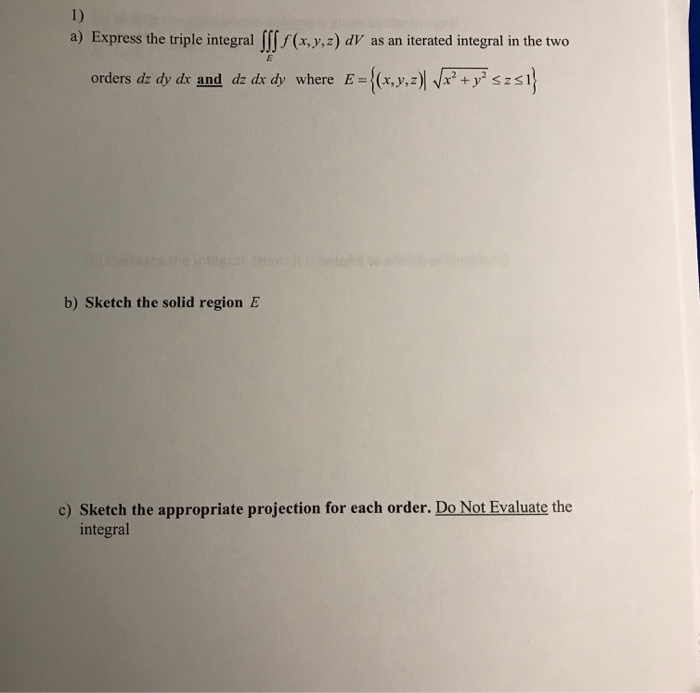please show all work in clean and legible handwriting with all labels and steps that is properly explained for PROBLEMS #1, 2, 3, AND 4. Any incorrect answers and not solving all 4 problems will get an immediate thumbs down because they did not follow directions, thank you 1) Express the triple integral Ⅲf (x,y,z) dV as an iterated integral in the two a) E={(x,y,z)Wr2+yszaj orders dzdy dr and dz dr dy where b) Sketch the solid region E c)...

• ### please check your answer Question Details Let W be the solid in the first octant bounded by the top half of the cyl...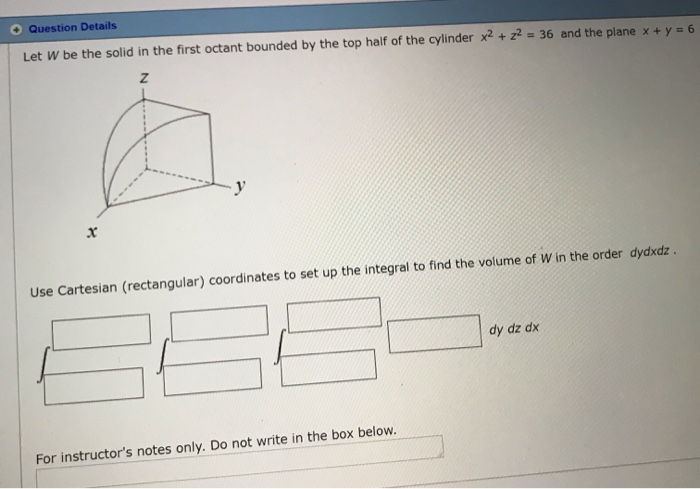please check your answer Question Details Let W be the solid in the first octant bounded by the top half of the cylinder x2 +z2= 36 and the plane x + y = 6 y Use Cartesian (rectangular) coordinates to set up the integral to find the volume of W in the order dydxdz. dy dz dx For instructor's notes only. Do not write in the box below. Question Details Let W be the solid in the first octant bounded...

• ### QUESTION 3 [ JJ dsdydr. Hence compute Sketch the solid whose volume is given by the integral (8 Marks) the volume u...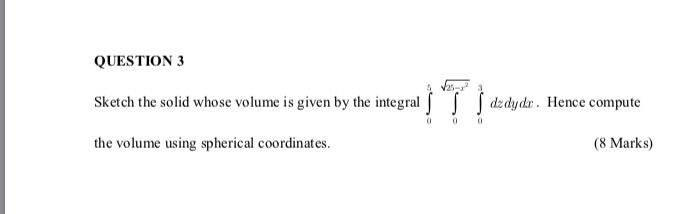QUESTION 3 [ JJ dsdydr. Hence compute Sketch the solid whose volume is given by the integral (8 Marks) the volume using spherical coordinates

• ### 3 D Question 3 5 pts Set up a triple integral for the volume of the...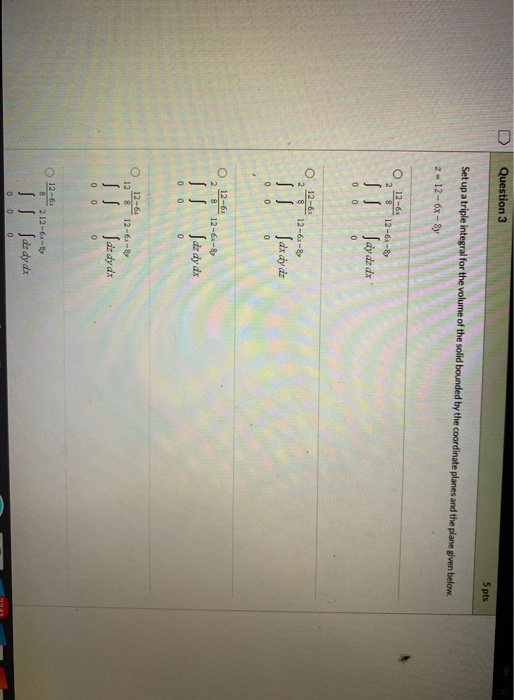3 D Question 3 5 pts Set up a triple integral for the volume of the solid bounded by the coordinate planes and the plane given below. 2-12 - 6x - Sy 0 12-63 2 812-6- I Savazax 0 0 0 012-6 28 12-6x By Sazdy dz 0 0 0 012-62 28 12-6x By Jdz dy dx 0 0 0 12-6 128 12-6x- Lazdy az 0 0 0 12-63 8 212-6-8 I dz dy dx 0 0